Courses

# Indeterminate Trusses and Industrial Frames (Part - 1) Civil Engineering (CE) Notes | EduRev

## Civil Engineering (CE) : Indeterminate Trusses and Industrial Frames (Part - 1) Civil Engineering (CE) Notes | EduRev

The document Indeterminate Trusses and Industrial Frames (Part - 1) Civil Engineering (CE) Notes | EduRev is a part of the Civil Engineering (CE) Course Structural Analysis.
All you need of Civil Engineering (CE) at this link: Civil Engineering (CE)

Instructional Objectives:

After reading this chapter the student will be able to

1. Make suitable approximations so that an indeterminate structure is reduced to a determinate structure.
2. Analyse indeterminate trusses by approximate methods.
3. Analyse industrial frames and portals by approximate methods.

Introduction

Force method of analysis is applied to solve indeterminate beams, trusses and frames. Displacement based methods are discussed for the analysis of indeterminate structures. These methods satisfy both equation of compatibility and equilibrium. Hence they are commonly referred as exact methods. It is observed that prior to analysis of indeterminate structures either by stiffness method or force method; one must have information regarding their relative stiffnesses and member material properties. This information is not available prior to preliminary design of structures. Hence in such cases, one can not perform indeterminate structural analysis by exact methods. Hence, usually in such cases, based on few approximations (which are justified on the structural behaviour under the applied loads) the indeterminate structures are reduced into determinate structures. The determinate structure is then solved by equations of statics. The above procedure of reducing indeterminate structures into determinate and solving them using equations of statics is known as approximate method of analysis as the results obtained from this procedure are approximate when compared to those obtained by exact methods. Also, approximate methods are used by design engineers to detect any gross error in the exact analysis of the complex structures. Depending upon the validity of assumptions, the results of approximate methods compare favourably with exact methods of structural analysis.

In some way, all structural methods of analysis are approximate as the exact loading on the structure, geometry; the material behaviour and joint resistance at beam column joints and soil-structure interaction are never known exactly. However, this is not a good enough reason for using approximate methods of analysis for the final design. After preliminary design, it is important to analyse the indeterminate structure by exact method of analysis. Based on these results, final design must be done. In this module both indeterminate industrial frames and building frames are analysed by approximate methods for both vertical and wind loads.

Indeterminate Trusses: Parallel-chord trusses with two diagonals in each panel.

Consider an indeterminate truss, which has two diagonals in each panel as shown in Fig. 35.1. This truss is commonly used for lateral bracing of building frames and as top and bottom chords of bridge truss.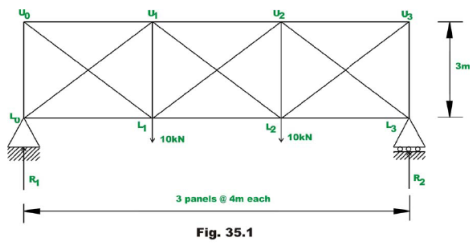This truss is externally determinate and internally statically indeterminate to 3rd degree. As discussed in lesson 10, module 2, the degree of static indeterminacy of the indeterminate planar truss is evaluated by

i = (m + r) - 2j        (reproduced here for convenience)

Where m, j and r respectively are number of members, joints and unknown reaction components. Since the given truss is indeterminate to 3rd degree, it is required to make three assumptions to reduce this frame into a statically determinate truss. For the above type of trusses, two types of analysis are possible.

1. If the diagonals are going to be designed in such a way that they are equally capable of carrying either tensile or compressive forces. In such a situation, it is reasonable to assume, the shear in each panel is equally divided by two diagonals. In the context of above truss, this amounts to 3 independent assumptions (one in each panel) and hence now the structure can be solved by equations of static equilibrium alone.

2. In some cases, both the diagonals are going to be designed as long and slender. In such a case, it is reasonable to assume that panel shear is resisted by only one of its diagonals, as the compressive force carried/resisted by the other diagonal member is very small or negligible. This may be justified as the compressive diagonal buckles at very small load. Again, this leads to three independent assumptions and the truss may be solved by equations of static alone.

Generalizing the above method, it is observed that one need to make n independent assumptions to solve nth order statically indeterminate structures by equations of statics alone. The above procedure is illustrated by the following examples.

Example 35.1
Evaluate approximately forces in the truss members shown in Fig. 35.2a, assuming that the diagonals are to be designed such that they are equally capable of carrying compressive and tensile forces.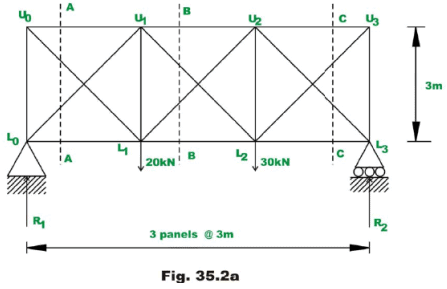Solution:
The given frame is externally determinate and internally indeterminate to order 3. Hence reactions can be evaluated by equations of statics only. Thus,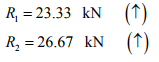(1)

Now it is required to make three independent assumptions to evaluate all bar forces. Based on the given information, it is assumed that, panel shear is equally resisted by both the diagonals. Hence, compressive and tensile forces in diagonals of each panel are numerically equal. Now consider the equilibrium of free body diagram of the truss shown left of A-A. This is shown in Fig. 35.2b.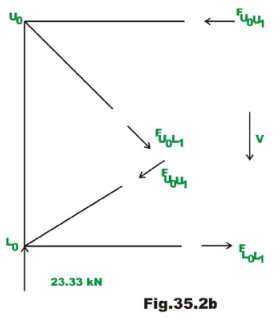For the first panel, the panel shear is 23.33 kN . Now in this panel, we have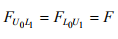(2)

Considering the vertical equilibrium of forces, yields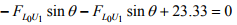(3)

2 sinθ = 23.33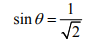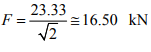(4)

Thus,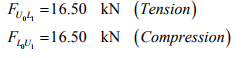Considering the joint , L0,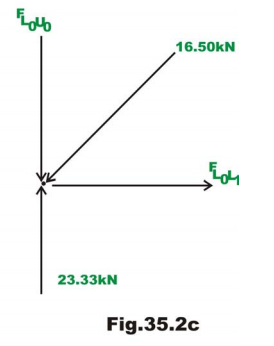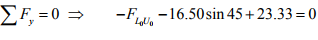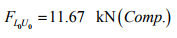(5)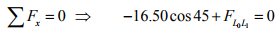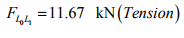(6)

Similarly,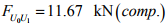Now consider equilibrium of truss left of section C−C (ref. Fig. 35.2d)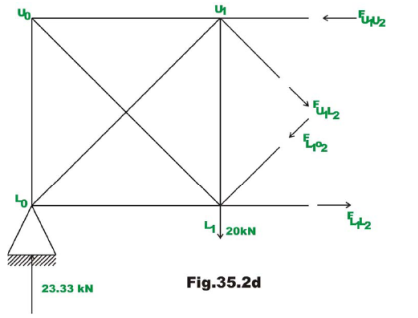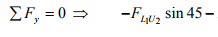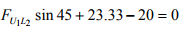(7)

It is given that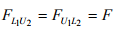2F sinθ = 3.33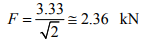Thus,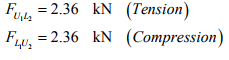Taking moment about U1 of all the forces,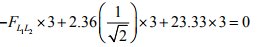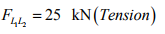(8)

Taking moment about L1 of all the forces,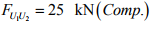(9)

Considering the joint equilibrium of L1 (ref. Fig. 35.2e),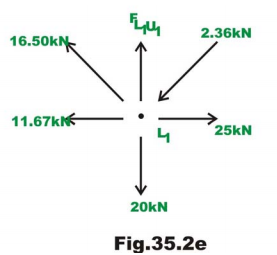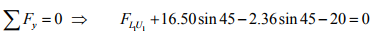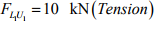(10)

Consider the equilibrium of right side of the section B − B (ref. Fig. 35.2f) the forces in the 3rd panel are evaluated.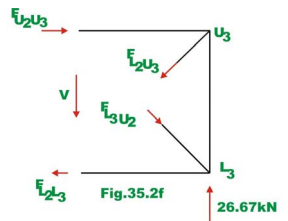We know that,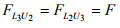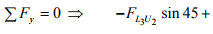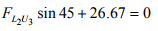(11)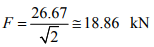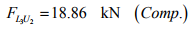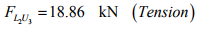(12)

Considering the joint equilibrium of L3 (ref. Fig. 35.2g), yields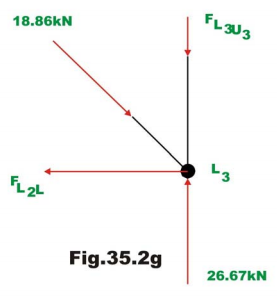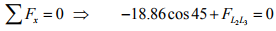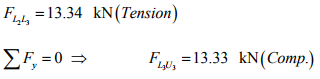The bar forces in all the members of the truss are shown in Fig. 35.2h. Also in the diagram, bar forces obtained by exact method are shown in brackets.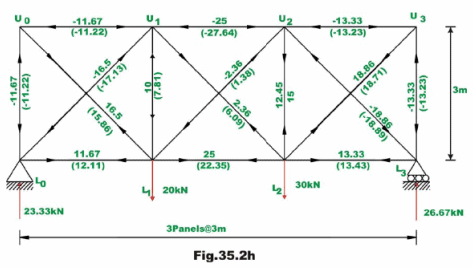Offer running on EduRev: Apply code STAYHOME200 to get INR 200 off on our premium plan EduRev Infinity!

## Structural Analysis

28 videos|114 docs|27 tests

,

,

,

,

,

,

,

,

,

,

,

,

,

,

,

,

,

,

,

,

,

;